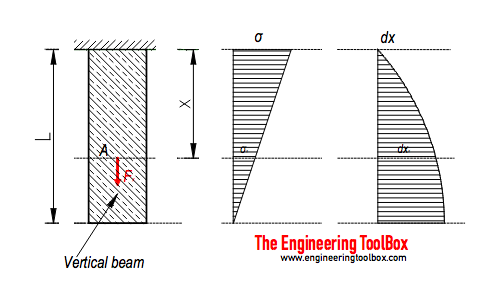Engineering ToolBox - Resources, Tools and Basic Information for Engineering and Design of Technical Applications!

# Weight of Beams - Stress and Strain

## Stress and deformation of vertical beams due to own weight.### Axial Force

The axial force acting in a cross sectional area in distance x in a vertical beam due to it's own weight - can be calculated as

Fx = γ  A (L - x)                                    (1)

where

Fx = axial force in distance x (N)

y = specific weight - unit volume weight (N/m3)

A = cross-sectional area (m2)

L = length of beam (m)

x = distance (m)

The specific weight can be expressed as

y = ρ g                                          (2)

where

ρ = density of beam (kg/m3)

g = acceleration of gravity (9.81 m/s2)

(1) can with (2) be modified to

Fxρ g  A (L - x)                            (2b)

### Axial Stress

The axial stress at a distance x can be calculated as

σx = Fx / A

= γ (L - x)

=  ρ g (L - x)                              (3)

where

σx = axial stress (Pa, N/m2)

Note! - the cross-sectional area is irrelevant.

The axial stress at distance x = L

σx=L = γ (L - L)

= ρ g (L - L)

= 0                                    (3a)

The axial stress at distance x = 0

σx=0 = γ (L - 0)

= ρ g (L - 0)

= γ L

= ρ g L                               (3b)

### Axial Deformation

The axial deformation at distance x can be calculated as

dxx = y x (2 L - x) / (2 E)

=   ρ g x (2 L - x) / (2 E)                           (4)

where

dx = deformation (m)

E = modulus of elasticity (N/m2)

The axial deformation at distance x = L

dxx=L = y L2 / (2 E)

= ρ g L2 / (2 E)                                (4a)

The axial deformation at distance x = 0

dxx=0 = 0                                    (4b)

### Example - Stress and Axial Deformation of a Vertical Steel Rod

A 45 m long steel rod with density 7280 kg/m3 and cross-sectional area 0.1 m2 is mounted as indicated in the figure above.

The maximum force acting in the rod at distance x = 0 m can be calculated with (1b)

Fx=0 (7280 kg/m3) (9.81 m/s2) (0.1 m2) ((45 m) - (0 m))

= 321376 N

= 321 kN

The maximum axial stress in the rod at distance x = 0 m can be calculated (3b)

σx=0 = (7280 kg/m3) (9.81 m/s2) (45 m)

= 3213756 N/m2 (Pa)

= 3.2 MPa

The modulus of elasticity for the steel rod is 200 GPa (200 109 N/m2).  The axial deformation at distance x = 45 m can be calculated with (4a)

dxx=45 = (7280 kg/m3) (9.81 m/s2) (45 m)2 / (2 (200 109 N/m2))

= 0.00036 m

= 0.4 mm

## Related Topics

• Mechanics - Forces, acceleration, displacement, vectors, motion, momentum, energy of objects and more.
• Beams and Columns - Deflection and stress, moment of inertia, section modulus and technical information of beams and columns.

## Engineering ToolBox - SketchUp Extension - Online 3D modeling!

Add standard and customized parametric components - like flange beams, lumbers, piping, stairs and more - to your Sketchup model with the Engineering ToolBox - SketchUp Extension - enabled for use with the amazing, fun and free SketchUp Make and SketchUp Pro .Add the Engineering ToolBox extension to your SketchUp from the SketchUp Pro Sketchup Extension Warehouse!

Translate

## Privacy

We don't collect information from our users. Only emails and answers are saved in our archive. Cookies are only used in the browser to improve user experience.

Some of our calculators and applications let you save application data to your local computer. These applications will - due to browser restrictions - send data between your browser and our server. We don't save this data.

## Citation

• Engineering ToolBox, (2016). Weight of Beams - Stress and Strain. [online] Available at: https://www.engineeringtoolbox.com/weight-beam-stress-strain-d_1937.html [Accessed Day Mo. Year].

Modify access date.

. .

#### Scientific Online Calculator3 10